﻿ 汉江中下游地区水资源多目标优化配置 Multi-Objective Optimal Allocation of Water Resources in the Middle and Lower Reaches of Hanjiang River Basin

Journal of Water Resources Research
Vol.07 No.03(2018), Article ID:24897,13 pages
10.12677/JWRR.2018.73025

Multi-Objective Optimal Allocation of Water Resources in the Middle and Lower Reaches of Hanjiang River Basin

Jing Tian1, Shenglian Guo1#, Dedi Liu1, Xingjun Hong1,2, Shaokun He1, Le Wang1,2

1State Key Laboratory of Water Resources and Hydropower Engineering Science, Wuhan University, Wuhan Hubei

2Changjiang Institute of Survey, Planning, Design and Research, Wuhan Hubei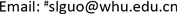Received: Apr. 22nd, 2018; accepted: May 9th, 2018; published: May 16th, 2018ABSTRACT

Combined with the principles of efficiency, fairness and sustainability in the water resources allocation, a multi-objective water resources optimal allocation model is proposed and applied in the middle and lower reaches of Hanjiang River basin. Taking economic, social and environment benefits as the objective functions, the non-dominated sorting genetic algorithm-II (NSGA-II) is used to optimize the allocation model. The years of 2010 and 2030 are selected as the baseline and planning level year, respectively. Based on the long-term historical runoff data from 1956 to 2011 and the water demand prediction in the planning level year, the optimal distribution is achieved by setting up the reservoir operation rules, water user priority and other variables. In the Pareto solutions, three indices of water shortage, economic benefit and pollutant discharge are compared and analyzed under different typical schemes, which indicates that the results are reasonable and applicable. This study can provide scientific basis for water resources planning and management in the middle and lower reaches of Hanjiang River basin, which help decision-makers achieve more efficient and accurate water resources allocation.

Keywords:Water Resources, Multi-Objective, Optimal Allocation, NSGA-II, Hanjiang River

1武汉大学水资源与水电工程科学国家重点实验室，湖北 武汉

2长江勘测规划设计研究有限责任公司，湖北 武汉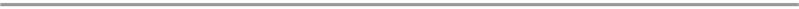1. 引言

2. 研究方法

2.1. 需水预测模型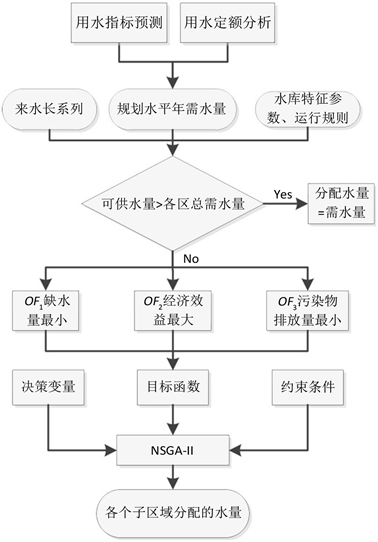Figure 1. Framework of the water resources optimal allocation model

$W{D}_{i,j}^{t}={q}_{i,j}^{t}\cdot {A}_{i,j}^{t}$ (1)

$E{T}_{0}=\frac{0.408\Delta \left({R}_{n}-G\right)+\gamma \frac{900}{T+273}{u}_{2}\left({e}_{a}-{e}_{d}\right)}{\Delta +\gamma \left(1+0.34{u}_{2}\right)}$ (2)

2.2. 水资源优化配置模型

2.2.1. 目标函数

$\mathrm{min}f\left(x\right)=\sum _{t=1}^{T}\sum _{i=1}^{n}\sum _{j=1}^{m}\left(W{D}_{i,j}^{t}-{x}_{i,j}^{t}\right)$ (3)

$\mathrm{max}f\left(x\right)=\sum _{t=1}^{T}\sum _{i=1}^{n}\sum _{j=1}^{m}\left(NE{R}_{i,j}\cdot {x}_{i,j}^{t}\right)$ (4)

$\mathrm{min}\left(TCOD\right)=\sum _{t=1}^{T}\sum _{i=1}^{n}\sum _{j=1}^{m}\left({d}_{i,j}\cdot {p}_{i,j}\cdot {x}_{i,j}^{t}\right)$ (5)

2.2.2 约束条件

1、分区水量平衡约束

${W}_{i}^{t}=\sum _{n=1}^{{N}_{i}}{\alpha }_{n,i}\cdot {W}_{n}^{t}+{R}_{i}^{t}+\sum _{k=1}^{{K}_{i}}{\beta }_{k,i}\cdot {O}_{k}^{t}-\sum _{j=1}^{m}\left(c{c}_{i,j}^{t}\cdot {x}_{i,j}^{t}\right)-{L}_{i}^{t}-T{W}_{i}^{t}$ (6)

2、水库约束

1) 水库水量平衡约束

${V}_{t+1}={V}_{t}+{I}_{t}-{O}_{t}-E{V}_{t}$ (7)

2) 水库库容约束

${V}_{\mathrm{min},t}\le {V}_{t}\le {V}_{\mathrm{max},t}$ (8)

3、需水约束

${x}_{i,j}^{t}\le W{D}_{i,j}^{t}$ (9)

4、供水能力约束

$\sum _{j=1}^{l}{x}_{i,j}^{t}\le AW{R}_{i}^{t}$ (10)

5、非负约束

${x}_{i,j}^{t}\ge 0$ (11)

2.2.3. 求解的优化算法

3. 汉江中下游水资源优化配置

3.1. 研究区域

3.2. 研究数据

1、水平年及计算步长

2、径流过程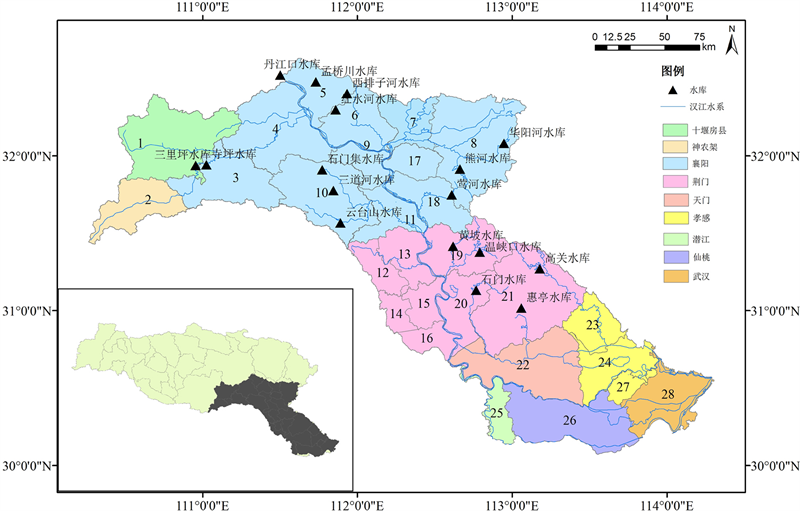Figure 2. Spatial distribution of the computing units in the middle and lower reaches of Hanjiang River basin

3、需水过程

4、水利工程数据

5、模型参数

1) 经济效益系数

2) 污水排放系数

3) 算法主要参数Table 1. Characteristics of reservoirs located in the middle and lower reaches of Hanjiang River basin

4. 结果分析与讨论

4.1. 需水预测结果Table 2. The economic return of each industry in the middle and lower reaches of Hanjiang River basin (yuan/m³)Table 3. Annual off-stream water demand projection of each city in the present and planning years

1) 汉江中下游地区及其各子区的河道外总需水量呈增加的趋势，在50%、75%、90%和95%四种频率下，2010水平年的河道外的毛需水量分别为103.57亿m3、110.94亿m3、117.82亿m3和122.91亿m3；2030水平年的河道外的毛需水量分别为110.94亿m3、117.12亿m3、123.79亿m3和127.97亿m3

2) 在同一水平年下，各用水部门需水中，以农业灌溉需水量最大，农业灌溉需水量随来水频率的增长呈增加的趋势；规划水平年的城镇生活和农村生活需水有小幅度的增加，城镇工业需水有大幅度的增加；在同一需水频率下，规划水平年的农业灌溉需水量相较于现状水平年呈减小趋势，占总需水量的比例也呈降低趋势。

3) 各个子区中，从三级套市的统计角度来看，襄阳市的需水量最大，其次是荆门市，神农架市的需水量最小。

4.2. 水资源优化配置结果

5. 结论(a) 缺水量和经济效益之间呈负相关的关系(b) COD的排放量和经济效益之间呈正相关的关系(c) COD的排放量和缺水量之间呈负相关的关系

Figure 3. Relation between objectives of the Pareto frontier for the water allocation modelTable 4. Three typical schemes for water allocation of multi-objective optimizationTable 5. The allocation results of three typical schemes

1) 在同一水平年下，汉江中下游地区的需水总量随来水频率的增长呈增加的趋势；在同一需水频率下，规划水平年的需水量相较于现状水平年呈增加的趋势。

2) 利用NSGA-II算法求解汉江中下游地区多目标水资源优化配置模型，能够得到区域的非劣解集，从解集的趋势可以看出：三个目标函数之间互相影响并且相互冲突。在三种典型分配方案下，各子区的缺水部门较为一致；规划水平年的水资源缺口均大于现状水平年。

3) 优化配置模型得到的结果可以为水资源管理和水污染控制提供支持。三种典型分配方案下，方案A与方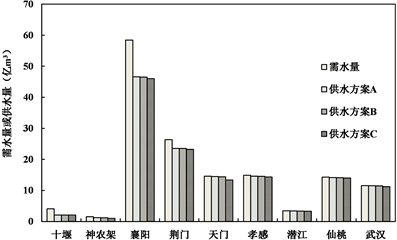(a) 2010水平年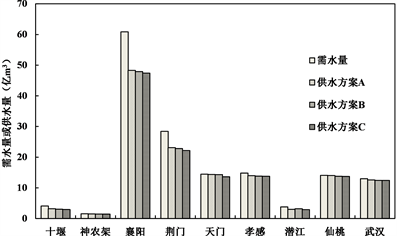(b) 2030水平年

Figure 4. The total amount of water supply in each city under three typical allocation schemes

 马建华. 关于汉江流域实施水量分配管理若干问题的思考[J]. 人民长江, 2010, 41(17): 1-6. MA Jianhua. Consideration on water quantity allocation in Hanjiang River Basin. Yangtze River, 2010, 41(17): 1-6. (in Chinese)

 韩雁. 基于区间不确定性水资源复杂系统协调演化研究[J]. 水利学报, 2008, 39(Z2): 892-898. HAN Yan. Study on coordination evolvement of complex water resources system with interval uncertainty. Shuilixuebao, 2008, 39(Z2): 892-898. (in Chinese)

 王峰, 李树荣, 杨亭亭. 区域水资源区间多目标规划模型及求解[J]. 水电能源科学, 2011, 29(4): 35-37. WANG Feng, LI Shurong and YANG Tingting. Regional water resource interval multi-objective programming model and its solution. Water Resources and Power, 2011, 29(4): 35-37. (in Chinese)

 刘德地, 郭生练, 郭海晋, 等. 实施最严格水资源管理制度面临的技术问题与挑战[J]. 水资源研究, 2014(3): 179-188. LIU Dedi, GUO Shenglian, GUO Haijing, et al. Technique controversies and challenges of applying the strictest water resources control system. Journal of Water Resources Research, 2014(3): 179-188. (in Chinese)

 HONG, X., GUO, S., WANG, L., et al. Evaluating water supply risk in the middle and lower reaches of Hanjiang River basin based on an integrated optimal water resources allocation model. Water, 2016, 8(9): 364.

 李维乾, 解建仓, 李建勋, 等. 基于灰色理论及改进类电磁学算法的水资源配置[J]. 水利学报, 2012, 43(12): 1447-1456. LI Weiqian, XIE Jiancang, LI Jianxun, et al. Water resources allocation based on the grey theory and the improved electromagnetism-like algorithm. Shuilixuebao, 2012, 43(12): 1447-1456. (in Chinese)

 尼庆伟, 杨凤林, 吴文业, 等. 康平县水资源配置大系统多目标模型[J]. 大连理工大学学报, 2009, 49(3): 340-344. NI Qingwei, YANG Fenglin, WU Wenye, et al. A large-scale system multi-objective programming model for water resources allocation in Kangping County. Journal of Dalian University of Technology, 2009, 49(3): 340-344. (in Chinese)

 余美, 芮孝芳. 宁夏银北灌区水资源优化配置模型及应用[J]. 系统工程理论与实践, 2009, 29(7): 181-192. YU Mei, RUI Xiaofang. Model of water resources optimal allocation and application in the Yinbei irrigation district of Ningxia. Systems Engineering-Theory & Practice, 2009, 29(7): 181-192. (in Chinese)

 刘德地, 王高旭, 陈晓宏, 等. 基于混沌和声搜索算法的水资源优化配置[J]. 系统工程理论与实践, 2011, 31(7): 1378-1386. LIU Dedi, WANG Gaoxu, CHEN Xiaohong, et al. Optimal water resources deployment based on chaos harmony search algorithm. Systems Engineering-Theory & Practice, 2011, 31(7): 1378-1386. (in Chinese)

 娄帅, 王慧敏, 牛文娟, 等. 基于免疫遗传算法水资源配置多阶段群决策优化模型研究[J]. 资源科学, 2013, 35(3): 569-577. LOU Shuai, WANG Huimin, NIU Wenjuan, et al. Optimization of multi-stage group decision making for water resource allocation. Resources Science, 2013, 35(3): 569-577. (in Chinese)

 BREKKE, L., LARSEN, M. D., AUSBURN, M. and TAKAICHI, L. Suburban water demand modeling using stepwise regression. Journal of the American Water Works Association, 2002, 94: 65-95. https://doi.org/10.1002/j.1551-8833.2002.tb09558.x

 ALLEN, R. G., PEREIRA, L. S., RAES, D., et al. Crop evapotranspiration: Guidelines for computing crop water requirements. Rome: FAO Irrigation and Drainage Paper 56, 1998.

 TENNANT, D. L. Instream flow regimens for fish, wildlife, recreation and related environmental resources. Fisheries, 1976, 1: 6-10. https://doi.org/10.1577/1548-8446(1976)001<0006:IFRFFW>2.0.CO;2

 刘士明, 于丹. 基于第二代非支配排序遗传算法(NSGA-Ⅱ)的水资源优化配置[J]. 水资源与水工程学报, 2013, 24(5): 185-188. LIU Shiming, YU Dan. Optimal allocation of water resources based on non-dominated sorting genetic algorithm-II. Journal of Water Resources & Water Engineering, 2013, 24(5): 185-188. (in Chinese)

 张超勇, 董星, 王晓娟, 等. 基于改进非支配排序遗传算法的多目标柔性作业车间调度[J]. 机械工程学报, 2010, 46(11): 156-164. ZHANG Chaoyong, DONG Xing, WANG Xiaojuan, et al. Improved NSGA-II for the multi-objective flexible job-shop scheduling problem. Journal of Mechanical Engineering, 2010, 46(11): 156-164. (in Chinese) https://doi.org/10.3901/JME.2010.11.156

 TABARI, M. M. R., SOLTANI, J. Multi-objective optimal model for conjunctive use management using SGAs and NSGA-II models. Water Resources Management, 2013, 27(1): 37-53. https://doi.org/10.1007/s11269-012-0153-7